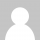# 编程改革

Codekana 这两个开发工具。

(顺便提一下，当遇到了多线程程序时，这种情况会恶化1000倍——不是变得更好，而是更坏。)

“我越用静态代码分析来检查代码，我越发现计算机的强大之处。”

### 一种观点

```def bubble_sort( input, output ):
swapped = True
while swapped:
swapped = False
for i = 1 to length(output) - 1:
if output[i+1] > output[i]:
swap( output[i+1], output[i] )
swapped = True```

```def sort(input[]) = output[]:
one_to_one_equal(input, output)
foreach i, j in 1..len(output):
where i < j:
output[i] <= output[j]```

• 首先，编译器如何能完成这个任务？真的有这种可能吗？在将来的文章里，我将会告诉你这是可能的，真的可能，编译器甚至能知道采用什么样的算法来获得这样的结果。
• 第二个问题是，如果把它应用到更复杂的情况中？我还是能向你展示，这种方式完全可以应用到任何的所有的编程和计算任务中，它只是一种更简单，更有效，更能避免错误的编程方式！

### 尾注

```   def one_to_one_equal(output[], input[]) = c:
c = relations(input[i], output[j])
foreach x = input[i]:  len(c(x,)) = 1
foreach x = output[i]: len(c(,x)) = 1
foreach x(a,b)=c[i]: a == b```

[英文原文：I want to fix programming ]

### 6 Responses to 编程改革

1.aomo says:

指令式和声明式的区别。。。。

2.Jerry Chou says:

没有本质差别，即使从直觉上来说陷阱也很多。

3.alex says:

我是有多痛恨程序。。
这篇文章我都不想看完。

4.select says:

感觉不到多特别之处。

5.依云 says:

让这种编译方式等待量子计算机出现吧。

6.林光海 says:

思路很新颖，文章得观点也很赞同，可以去尝试一下，纯当玩了